## How To Count the number of days, weeks, months or years between two dates?

Have you ever considered calculating how many days, weeks, months or years between two given dates in Excel? This tutorial can help you to finish the following operations as soon as possible.

#### Count the number of days between two dates with formulas

To count the number of days between two given dates, please use below formulas:

1. Enter any one of the below formulas into a blank cell where you want to get the result:

=DATEDIF(A2,B2,"D")
=B2-A2

Note: In the above formula, A2 is the start date cell and B2 is the end date cell.

2. Then, drag the fill handle down to the cells that you want to apply this formula, and the number of days have been calculated, see screenshot: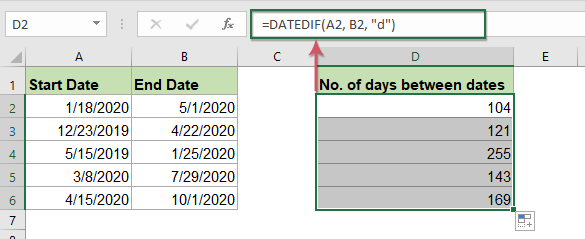#### Count the number of weeks between two dates with formulas

To count how many weeks between two dates, you can use the below handy formula, just subtract start date from the end date and divide by 7.

1. Enter any one of the below formulas into a blank cell:

=(DATEDIF(A2,B2,"D")/7)
=(B2-A2)/7

Note: In the above formula, A2 is the start date cell and B2 is the end date cell.

2. Then, drag the fill handle down to fill this formula, and you will get the weeks in decimal numbers, see screenshot: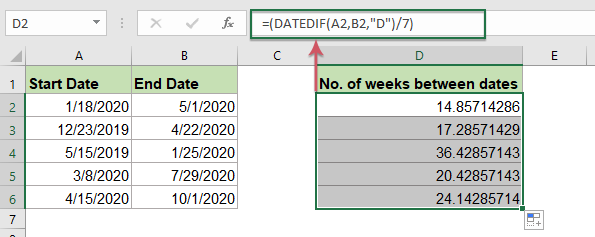• TipsIf you want to get the number of full weeks between two dates, please apply this formula:
• =ROUNDDOWN((DATEDIF(A2, B2, "d") / 7), 0)
=INT((B2-A2)/7)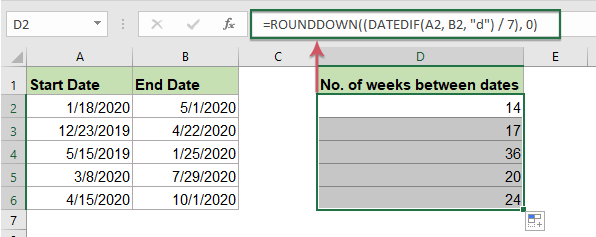Calculate Various Difference Between Two Dates In Days, Weeks, Months, Years etc.

Too many formulas to remember, with Kutools for Excel's Date & Time Helper feature, you can quickly get the various difference results between two dates based on your need without remembering any formulas, such as weeks + days，months + weeks and so on. Click to download Kutools for Excel!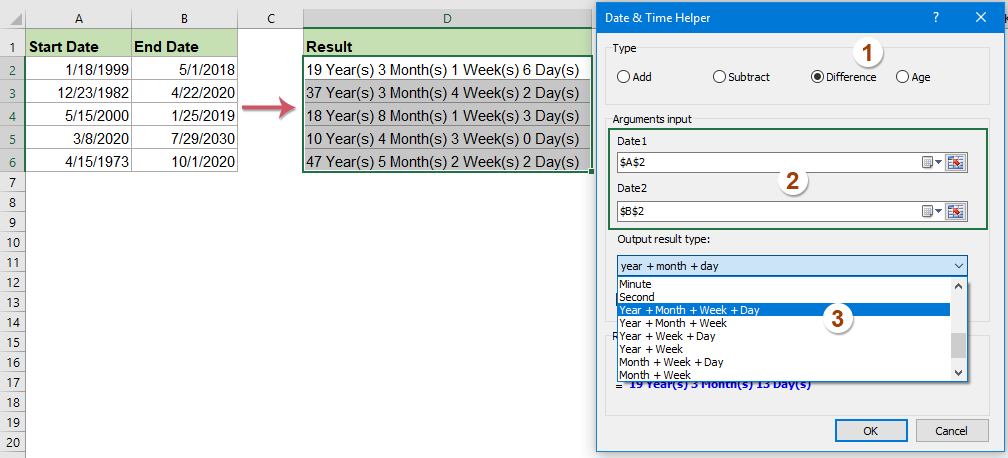Kutools for Excel: with more than 300 handy Excel add-ins, free to try with no limitation in 30 days. Download and free trial Now!

#### Count the number of months between two dates with formula

If you want to determine the number of months between two dates, you can use this DATEDIF function.

1. Please enter the following formula into a blank cell where you want to output the result:

=DATEDIF(A2,B2,"m")

Note: In the above formula, A2 is the start date cell and B2 is the end date cell.

2. Then, drag the fill handle down to fill this formula, and you will get the number of completed months between the given two dates, see screenshot: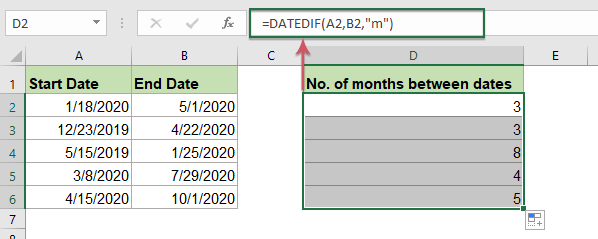• TipsIf you want to get the number of full weeks between two dates, please apply this formula:
• =DATEDIF(A2,B2,"m")&"months "&DATEDIF(A2,B2,"md")&"days"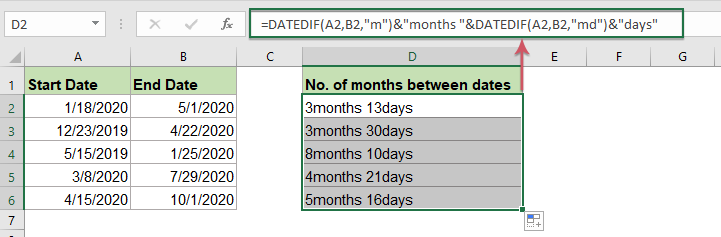#### Count the number of years between two dates with formula

With the above DATEDIF function, you can also calculate the number of years between two dates.

1. Apply the below formula into a blank cell:

=DATEDIF(A2,B2,"y")

Note: In the above formula, A2 is the start date cell and B2 is the end date cell.

2. Then, drag the fill handle down to the cells that you want to apply this formula, and all the number of years have been calculated as below screenshot shown: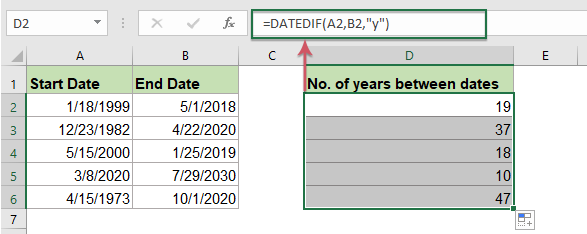#### Count the number of years months and days between two dates with formulas

Sometimes, you need to know exactly how many years, months and days between two dates, in this situation, the following long formula may help you.

1. In a blank cell, enter or copy the following formula:

=DATEDIF(A2, B2, "y") &" years "&DATEDIF(A2, B2, "ym") &" months " &DATEDIF(A2, B2, "md") &" days"

Note: In the above formula, A2 is the start date cell and B2 is the end date cell.

2. And then, drag the fill handle to fill this formula, and the specific number of years, months and days between the dates are calculated at once, see screenshot: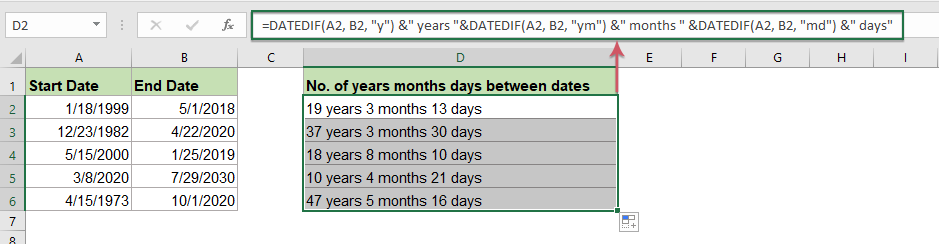#### Calculate various difference between two dates in days, weeks, months, years with a powerful feature

May be, you are annoying with the above formulas, if you have Kutools for Excel, with its Date & Time Helper feature, you can quickly get the various difference results between two dates based on your need, such as weeks + days，months + weeks and so on.

Note:To apply this Date & Time Helper, firstly, you should download the Kutools for Excel, and then apply the feature quickly and easily.

After installing Kutools for Excel, please do as this:

1. Click a cell where you want to locate the result, and then click KutoolsFormula Helper > Date & Time helper, see screenshot: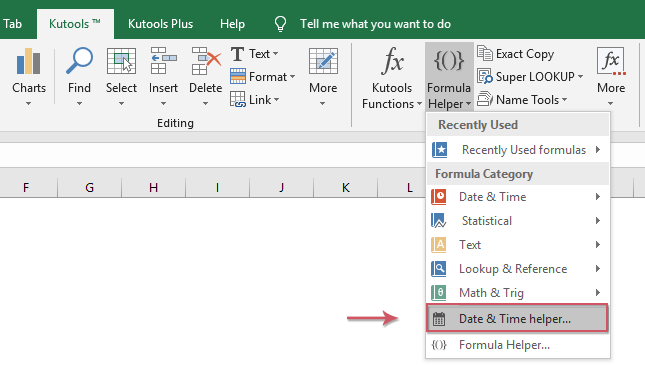2. In the Date & Time Helper dialog box, do the following operations:

• Check Difference option from the Type section;
• In the Arguments input textboxes, select the cells which contain the start date and end date separately;
• Then, choose the output result type as you need. For example, if you want to get the number of years, months, weeks and days between two dates, you just select Year + Month + Week + Day.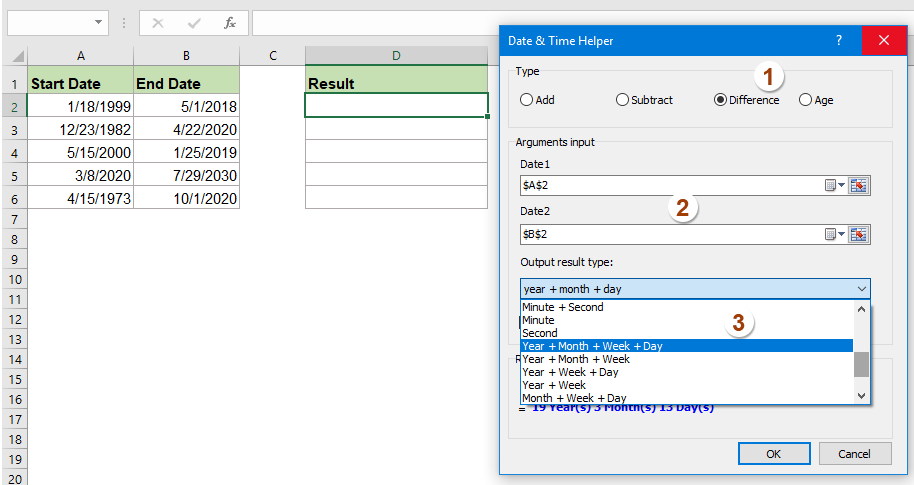3. After finishing the settings, please click OK button, and you will get the first calculated result, then, you just need to drag the fill handle down to the cells that you want to apply this formula, and you will get the following result as you need: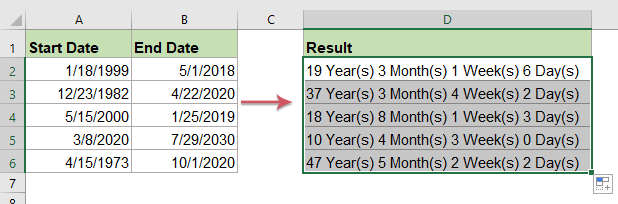#### More relative date and time articles:

• Calculate Hours Between Times After Midnight In Excel
• Supposing you have a time table to record your work time, the time in Column A is the start time of today and time in Column B is the end time of the following day. Normally, if you calculate the time difference between the two times by directly minus "=B2-A2", it will not display the correct result as left screenshot shown. How could you calculate the hours between two times after midnight in Excel correctly?
• Count The Number Of Days / Workdays / Weekends Between Two Dates
• Have you ever needed to count the number of days between two dates in Excel? May be, sometimes, you just only want to calculate the workdays between two dates, and sometime, you need to count the weekend days only between the two dates. How could you count the number of days between two dates in a certain condition?

### Best Office Productivity Tools

 Popular Features: Find, Highlight or Identify Duplicates   |  Delete Blank Rows   |  Combine Columns or Cells without Losing Data   |   Round without Formula ... Super Lookup: Multiple Criteria VLookup  |   Multiple Value VLookup  |   VLookup Across Multiple Sheets   |   Fuzzy Lookup .... Advanced Drop-down List: Quickly Create Drop Down List   |  Dependent Drop Down List   |  Multi-select Drop Down List .... Column Manager: Add a Specific Number of Columns  |  Move Columns  |  Toggle Visibility Status of Hidden Columns  |  Compare Ranges & Columns ... Featured Features: Grid Focus   |  Design View   |   Big Formula Bar   |  Workbook & Sheet Manager   |  Resource Library (Auto Text)   |  Date Picker   |  Combine Worksheets   |  Encrypt/Decrypt Cells   |  Send Emails by List   |  Super Filter   |   Special Filter (filter bold/italic/strikethrough...) ... Top 15 Toolsets:  12 Text Tools (Add Text, Remove Characters, ...)   |   50+ Chart Types (Gantt Chart, ...)   |   40+ Practical Formulas (Calculate age based on birthday, ...)   |   19 Insertion Tools (Insert QR Code, Insert Picture from Path, ...)   |   12 Conversion Tools (Numbers to Words, Currency Conversion, ...)   |   7 Merge & Split Tools (Advanced Combine Rows, Split Cells, ...)   |   ... and more

Supercharge Your Excel Skills with Kutools for Excel, and Experience Efficiency Like Never Before. Kutools for Excel Offers Over 300 Advanced Features to Boost Productivity and Save Time.  Click Here to Get The Feature You Need The Most...#### Office Tab Brings Tabbed interface to Office, and Make Your Work Much Easier

• Enable tabbed editing and reading in Word, Excel, PowerPoint, Publisher, Access, Visio and Project.
• Open and create multiple documents in new tabs of the same window, rather than in new windows.
• Increases your productivity by 50%, and reduces hundreds of mouse clicks for you every day!No ratings yet. Be the first to rate!
This comment was minimized by the moderator on the site
Dateif formula how to input?
This comment was minimized by the moderator on the site
Hi I am trying to build up a salary report where i need to split a person salary by month if they completed that full month , and if their start or end date is after the beginning or the did of the month then calculate that month based on the network days worked. the problem I am getting when using the theses date formulas is that are not showing the correct completed month only. for example.

Start Date End Date
07/09/2022 11/01/2023

this should be 3 months and 26 days (18 working days in September and 8 working days in Jan 2023) = DatedIf formula is showing 4
Can anyone help?

thanks,
Charlotte
This comment was minimized by the moderator on the site
Quisiera saber, si podría calcular cuántos martes hay entre dos fechas; por ejemplo, en tres años o no es posible
Gracias de antemano por su colaboración
This comment was minimized by the moderator on the site
Hello, Pilar,
To get the number of a specific weekday, you can apply the below formula:
``=SUMPRODUCT(--(WEEKDAY(ROW(INDIRECT(A2&":"&B2)))=3))``

Note: A2 is the start date, B2 is the end date, and the number 3 indicates Tuesday, 1 to 7 indicates from Sunday to Saturday, you can change the number to your need.

This comment was minimized by the moderator on the site
Muchísimas gracias por su ayuda
This comment was minimized by the moderator on the site
Love Kutools! I purchased years ago and still visit the extednoffice.com site when I need fast solutions!
This comment was minimized by the moderator on the site
Avenger vs Marvell
perhitungan permainan roulette
perhitungan permainan sicbo
This comment was minimized by the moderator on the site
How can obtain age from two DOB. Eg- i need to calculate the age from DATE of Birth till the Date of Treatment was started
This comment was minimized by the moderator on the site
how to obtain matching no of months between two sets of dates ?
This comment was minimized by the moderator on the site
What is the formula for 01/05/2017 13:01:20 minus 01/05/2017 08:59:27 to get the total hours.
This comment was minimized by the moderator on the site
I need the following and cannot figure out the formula I have been given the date of birth, date of exam. I need a third date, (record purge date) that has the following rules; IF they are 18 or over by exam date, the purge date will be 7 years from exam date IF they are under 18 by exam date, the purge date would be 7 years from when they turn 18
This comment was minimized by the moderator on the site
Thank You so much for your way. 2017 calendar printable
There are no comments posted here yet